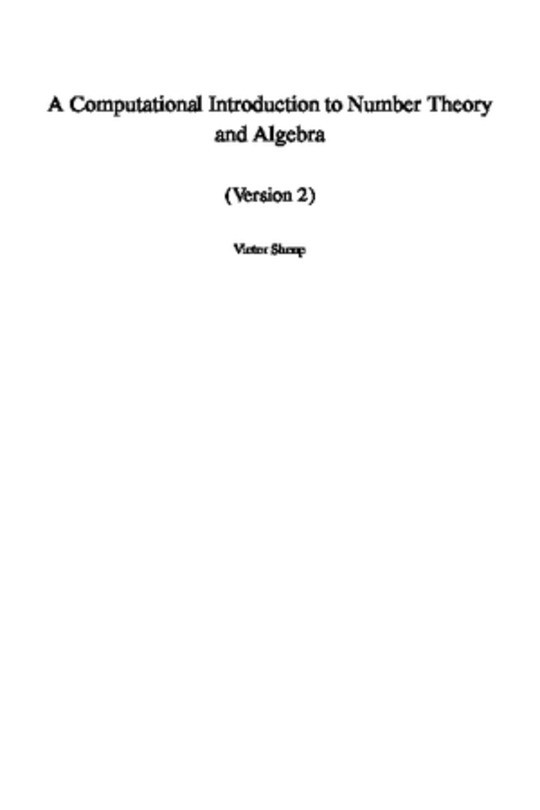Victor Shoup
A Computational Introduction to Number Theory and Algebra (Version 2)
Free
Description
Contents
Reviews
Language
English
ISBN
Unknown
Title
Contents
Preface
Preliminaries
1 Basic properties of the integers
1.1 Divisibility and primality
1.2 Ideals and greatest common divisors
1.3 Some consequences of unique factorization
2 Congruences
2.1 Equivalence relations
2.2 Definitions and basic properties of congruences
2.3 Solving linear congruences
2.4 The Chinese remainder theorem
2.5 Residue classes
2.6 Euler's phi function
2.7 Euler's theorem and Fermat's little theorem
2.9 Summations over divisors
3 Computing with large integers
3.1 Asymptotic notation
3.2 Machine models and complexity theory
3.3 Basic integer arithmetic
3.4 Computing in Zn
3.5 Faster integer arithmetic (*)
3.6 Notes
4 Euclid's algorithm
4.1 The basic Euclidean algorithm
4.2 The extended Euclidean algorithm
4.3 Computing modular inverses and Chinese remaindering
4.4 Speeding up algorithms via modular computation
4.5 An effective version of Fermat's two squares theorem
4.6 Rational reconstruction and applications
4.7 The RSA cryptosystem
4.8 Notes
5 The distribution of primes
5.1 Chebyshev's theorem on the density of primes
5.2 Bertrand's postulate
5.3 Mertens' theorem
5.4 The sieve of Eratosthenes
5.5 The prime number theorem …and beyond
5.6 Notes
6 Abelian groups
6.1 Definitions, basic properties, and examples
6.2 Subgroups
6.3 Cosets and quotient groups
6.4 Group homomorphisms and isomorphisms
6.5 Cyclic groups
6.6 The structure of finite abelian groups (*)
7 Rings
7.1 Definitions, basic properties, and examples
7.2 Polynomial rings
7.3 Ideals and quotient rings
7.4 Ring homomorphisms and isomorphisms
7.5 The structure of Zn*
8 Finite and discrete probability distributions
8.1 Basic definitions
8.2 Conditional probability and independence
8.3 Random variables
8.4 Expectation and variance
8.5 Some useful bounds
8.6 Balls and bins
8.7 Hash functions
8.8 Statistical distance
8.9 Measures of randomness and the leftover hash lemma (*)
8.10 Discrete probability distributions
8.11 Notes
9 Probabilistic algorithms
9.1 Basic definitions
9.2 Generating a random number from a given interval
9.3 The generate and test paradigm
9.4 Generating a random prime
9.5 Generating a random non-increasing sequence
9.6 Generating a random factored number
9.7 Some complexity theory
9.8 Notes
10 Probabilistic primality testing
10.1 Trial division
10.2 The Miller--Rabin test
10.3 Generating random primes using the Miller--Rabin test
10.4 Factoring and computing Euler's phi function
10.5 Notes
11 Finding generators and discrete logarithms in Zp*
11.1 Finding a generator for Zp*
11.2 Computing discrete logarithms in Zp*
11.3 The Diffie--Hellman key establishment protocol
11.4 Notes
12 Quadratic reciprocity and computing modular square roots
12.1 The Legendre symbol
12.2 The Jacobi symbol
12.3 Computing the Jacobi symbol
12.5 Computing modular square roots
12.7 Notes
13 Modules and vector spaces
13.1 Definitions, basic properties, and examples
13.2 Submodules and quotient modules
13.3 Module homomorphisms and isomorphisms
13.4 Linear independence and bases
13.5 Vector spaces and dimension
14 Matrices
14.1 Basic definitions and properties
14.2 Matrices and linear maps
14.3 The inverse of a matrix
14.4 Gaussian elimination
14.5 Applications of Gaussian elimination
14.6 Notes
15 Subexponential-time discrete logarithms and factoring
15.1 Smooth numbers
15.2 An algorithm for discrete logarithms
15.3 An algorithm for factoring integers
15.4 Practical improvements
15.5 Notes
16 More rings
16.1 Algebras
16.2 The field of fractions of an integral domain
16.3 Unique factorization of polynomials
16.4 Polynomial congruences
16.5 Minimal polynomials
16.6 General properties of extension fields
16.7 Formal derivatives
16.8 Formal power series and Laurent series
16.9 Unique factorization domains (*)
16.10 Notes
17 Polynomial arithmetic and applications
17.1 Basic arithmetic
17.2 Computing minimal polynomials in F[X]/(f) (I)
17.3 Euclid's algorithm
17.4 Computing modular inverses and Chinese remaindering
17.5 Rational function reconstruction and applications
17.6 Faster polynomial arithmetic (*)
17.7 Notes
18 Linearly generated sequences and applications
18.1 Basic definitions and properties
18.2 Computing minimal polynomials: a special case
18.3 Computing minimal polynomials: a more general case
18.4 Solving sparse linear systems
18.5 Computing minimal polynomials in F[X]/(f) (II)
18.6 The algebra of linear transformations (*)
18.7 Notes
19 Finite fields
19.1 Preliminaries
19.2 The existence of finite fields
19.3 The subfield structure and uniqueness of finite fields
19.4 Conjugates, norms and traces
20 Algorithms for finite fields
20.1 Tests for and constructing irreducible polynomials
20.2 Computing minimal polynomials in F[X]/(f) (III)
20.3 Factoring polynomials: square-free decomposition
20.4 Factoring polynomials: the Cantor--Zassenhaus algorithm
20.5 Factoring polynomials: Berlekamp's algorithm
20.6 Deterministic factorization algorithms (*)
20.7 Notes
21 Deterministic primality testing
21.1 The basic idea
21.2 The algorithm and its analysis
21.3 Notes
Appendix: Some useful facts
Bibliography
Index of notation
Index
The book hasn't received reviews yet.